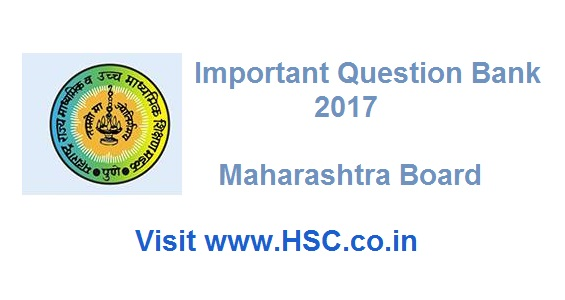# Mathematics & Statistics  – Important Question Bank for Maharashtra HSC Board Exam 2017

HSC Board Exams are fast approaching and students are getting anxious about how to prepare for their HSC Board Exams. So we had mentioned some HSC Study Tips to help students in Cracking HSC Exams.

We have also created Free Mock Test of MCQ- Multi Choice Question for students to help them prepare for the exam like scenario.

After the tremendous success of our last year Important Questions Bank for Maharashtra HSC Board Exam 2016, we have also created a list of Most Important Question Bank for Maharashtra HSC Board Exam 2017 which are likely to appear in HSC Board Exams this year.To unlock the content Click on any of 3 buttons available in the box below saying “This content is Locked”. Once you click on the button the content will get unlocked on same page itself. You must click on social media button showing in below box ie Facebook, Twitter or Google Plus to unlock the content.

Hi, we’re trying to collate and gather the data and would be updating it here a few days before the exam. Please keep on visiting our website for updates.

Please use the comments box below and post questions that you think are important from your analysis. It would help the HSC community a lot.

Do subscribe to our updates so that you do not miss out on any important information that we push your way.

1. The probability that a certain kind of component will survive a check test is 0.6. Find the probability that exactly 2 of the next 4 tested components survive.
2. Find the approximate value of tan – 1 (1.0001).
3. Find the volume of the solid generated, when the area between ellipse 4x2 + 9y2 = 36 and the chord AB, with A = (3,0), B = (0,2); is revolved about X-axis.
4. Find the area of the region common to the circle x 2 + y 2 = 9 and the parabola y2 = 8x
5. The surface area of a spherical balloon is increasing at the rate of 2 cm2/sec. At what rate is the volume of the balloon is increasing when the radius of the balloon is 6 cm?
6. A random variable X has the following probability distribution:
 X =  x 0 1 2 3 4 5 6 P(X=x) K 3k 5 k 7k 9k 11k 13k

(a) Find k, (b) Find P (0<X<4)

(c) Obtain cumulative distribution function (c.d.f) of X.

1. Verify Rolle ’s Theorem for the function: f(x) = x2 – 5x + 9 on [1, 4]
2. If A,B,C,D are (1,1,1), (2,1,3), (3,2,2), and (3,3,4) respectively, then find the volume of the parallelepiped with AB, AC and AD are concurrent edges.
3. In any ΔABC, with usual notations, prove that b2 = c2 + a2 – 2ca cos B.
4. A baker man sells 5 types of cakes. Profits due to the sale of each type of cake are respectively Rs 3, Rs 2.5, Rs 2, Rs 1.5, Rs 1. The demands for these cakes are 10%, 5%, 25%, 45% and 15% respectively. What is the expected profit per cake?
5. A point source of light is hung 30 feet directly above a straight horizontal path on which a man of 6 feet in height is walking. How fast will the man’s shadow lengthen and how fast will the tip of shadow move when he is walking away from the light at the rate of 100 ft/min.
6. Solve: 3ex tan ydx + (1 + ex) sec2 ydy = 0, also find the particular solution when x = 0 and y = π
7. Amit and Rohit started a business by investing Rs 20,000 each. After 3 months Amit withdrew Rs 5,000 and Rohit put in Rs 5,ooo additionally. How should a profit of Rs 12.800 be divided between them at the end of the year?
8. Find the present value of an annuity immediate Rs 18,000 p.a for 3 years at 9% p.a. compounded annually. [Given (1.09)-3 = 0.7722]
9. Using the truth table, examine whether the statement pattern (p →q) ↔ (~ p v q) is an tautology, a contradiction or a contingency.
10. Discuss extreme value of the function f(x) = x log x
11. A card is drawn at random and replaced four times from a well shuffled pack of 52 cards. Find the probability that-a) Tow diamond cards are drawn b) At least one diamond card is drawn.
12. Find the agent’s commission at 15% on the first premium if he places insurance for Rs. 2,000,000 on the life of a person, the premium being at the rate of Rs. 35 per thousand, per annum, paid annually.
13. A banker’s discount calculated for 1 years is 26 times his gain. Find the rate of interest.
14. Examine whether the following statement (p ^ q) v ( ~p v ~ q ) is a tautology or contradiction or neither of them.

Don’t forget to read : MUST REMEMBER THINGS on the day of Exam for HSC Students

Maharashtra HSC Board Exam – Important Question Bank 2017

## 8 thoughts on “Mathematics & Statistics  – Important Question Bank for Maharashtra HSC Board Exam 2017”

1.Clairila says:

Please give some more important questions for Maths and Biology subjects.

2.XAVIER says:

PLEASE GIVE ME IMPORTANT QUESTIONS OF HINDI ?

3.kiran says:

Hello,
HSC Board,
i got the few mathes question of ur updates,
i want to more questions of mathematics paper 1 & 2,
im request
4.Sunil says:
5.MOHAMMAD says:
6.Ketan says: